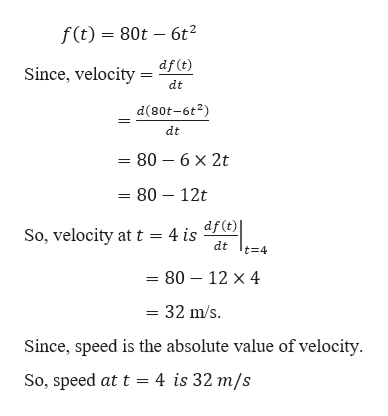2.1Can you show me the steps to solve this problem?Directions: A particle moves along a straight line with equation of motion s = f(t), where s is measured in meters and t in seconds. Find the velocity and the speed when t = 4.43) f(t) = 80t - 6t^2

Question

2.1

Can you show me the steps to solve this problem?

Directions: A particle moves along a straight line with equation of motion s = f(t), where s is measured in meters and t in seconds. Find the velocity and the speed when t = 4.

43) f(t) = 80t - 6t^2

Step 1

Given,

...help_outlineImage Transcriptionclosef(t) 80t 6t df (t) Since, velocity dt d(80t-6t2) dt 80 6 x 2t = 80 - 12t So, velocity att = 4 is dF (t) t 4 dt = 80 12 x 4 32 m/s = Since, speed is the absolute value of velocity So, speed at t 4 is 32 m/s fullscreen

Want to see the full answer?

See Solution

Want to see this answer and more?

Our solutions are written by experts, many with advanced degrees, and available 24/7

See Solution
Tagged in

Derivative